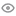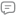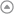New update is available. Click here to update.Chandigarh University 2025
My StatsEXP gained9715Level
6 (Specialist)
Community statsDiscussions
00
Know more614
Total problems solved
594
Easy
19
Moderate
1
Hard
0
NinjaCurrent streak:

44 days

Longest streak:

44 days

Less

More

Achievements2
Ronin
Topics
Arrays
Discussions
C++ Easy and simple method
Interview problems

#include <bits/stdc++.h>

string convertString(string str)

{

str=toupper(str);

for(int i = 0; i<str.length(); i++){

if(str[i-1] == ' '){

str[i] = toupper(str[i]);

}

}

return str;

}

1).The function convertString is declared, which takes a single string argument str.

2).It begins by changing the first character of the input string str to uppercase. This is done by calling the toupper function on the first character and assigning the result back to str.

3).The code then enters a for loop that iterates through the characters of the string str. The loop variable i starts at 0 and goes up to the length of the string minus one (i < str.length()).

4)Inside the loop, it checks if the character at index i (i.e., str[i]) is preceded by a space character (i.e., str[i-1] == ' '). If this condition is true, it means that the current character is the first character of a new word.

5)When the condition in step 4 is true, it calls the toupper function on the character at index i and assigns the result back to str[i]. This converts the character to uppercase.

6)The loop continues to the next character, and the process is repeated for the rest of the string.

7)After the loop finishes processing all characters in the string, the modified string is returned.

Krish chouhan
Published On 06-Nov-2023121 views0 repliesC++ Easy and simple method
Interview problems

#include <bits/stdc++.h>

string convertString(string str)

{

str=toupper(str);

for(int i = 0; i<str.length(); i++){

if(str[i-1] == ' '){

str[i] = toupper(str[i]);

}

}

return str;

}

1).The function convertString is declared, which takes a single string argument str.

2).It begins by changing the first character of the input string str to uppercase. This is done by calling the toupper function on the first character and assigning the result back to str.

3).The code then enters a for loop that iterates through the characters of the string str. The loop variable i starts at 0 and goes up to the length of the string minus one (i < str.length()).

4)Inside the loop, it checks if the character at index i (i.e., str[i]) is preceded by a space character (i.e., str[i-1] == ' '). If this condition is true, it means that the current character is the first character of a new word.

5)When the condition in step 4 is true, it calls the toupper function on the character at index i and assigns the result back to str[i]. This converts the character to uppercase.

6)The loop continues to the next character, and the process is repeated for the rest of the string.

7)After the loop finishes processing all characters in the string, the modified string is returned.

Krish chouhan
Published On 06-Nov-2023121 views0 repliesC++ Easy and simple method
Interview problems

#include <bits/stdc++.h>

string convertString(string str)

{

str=toupper(str);

for(int i = 0; i<str.length(); i++){

if(str[i-1] == ' '){

str[i] = toupper(str[i]);

}

}

return str;

}

1).The function convertString is declared, which takes a single string argument str.

2).It begins by changing the first character of the input string str to uppercase. This is done by calling the toupper function on the first character and assigning the result back to str.

3).The code then enters a for loop that iterates through the characters of the string str. The loop variable i starts at 0 and goes up to the length of the string minus one (i < str.length()).

4)Inside the loop, it checks if the character at index i (i.e., str[i]) is preceded by a space character (i.e., str[i-1] == ' '). If this condition is true, it means that the current character is the first character of a new word.

5)When the condition in step 4 is true, it calls the toupper function on the character at index i and assigns the result back to str[i]. This converts the character to uppercase.

6)The loop continues to the next character, and the process is repeated for the rest of the string.

7)After the loop finishes processing all characters in the string, the modified string is returned.

Krish chouhan
Published On 06-Nov-2023121 views0 repliesC++ Easy and simple method
Interview problems

#include <bits/stdc++.h>

string convertString(string str)

{

str=toupper(str);

for(int i = 0; i<str.length(); i++){

if(str[i-1] == ' '){

str[i] = toupper(str[i]);

}

}

return str;

}

1).The function convertString is declared, which takes a single string argument str.

2).It begins by changing the first character of the input string str to uppercase. This is done by calling the toupper function on the first character and assigning the result back to str.

3).The code then enters a for loop that iterates through the characters of the string str. The loop variable i starts at 0 and goes up to the length of the string minus one (i < str.length()).

4)Inside the loop, it checks if the character at index i (i.e., str[i]) is preceded by a space character (i.e., str[i-1] == ' '). If this condition is true, it means that the current character is the first character of a new word.

5)When the condition in step 4 is true, it calls the toupper function on the character at index i and assigns the result back to str[i]. This converts the character to uppercase.

6)The loop continues to the next character, and the process is repeated for the rest of the string.

7)After the loop finishes processing all characters in the string, the modified string is returned.

Krish chouhan
Published On 06-Nov-2023121 views0 repliesc++ easy and simple method to solve problem
Interview problems

vector<int> printDivisors(int n) {

vector<int> ans;

for(int i = 1; i <= sqrt(n); i++) {

if(n % i == 0) {

ans.push_back(i);

int div = n / i;

if(n % div == 0 and i != div)

ans.push_back(div);

}

}

sort(ans.begin(), ans.end());

return ans;

}

Krish chouhan
Published On 03-Nov-2023300 views0 repliesc++ easy and simple method to solve problem
Interview problems

vector<int> printDivisors(int n) {

vector<int> ans;

for(int i = 1; i <= sqrt(n); i++) {

if(n % i == 0) {

ans.push_back(i);

int div = n / i;

if(n % div == 0 and i != div)

ans.push_back(div);

}

}

sort(ans.begin(), ans.end());

return ans;

}

Krish chouhan
Published On 03-Nov-2023300 views0 repliesc++ easy and simple method to solve problem
Interview problems

vector<int> printDivisors(int n) {

vector<int> ans;

for(int i = 1; i <= sqrt(n); i++) {

if(n % i == 0) {

ans.push_back(i);

int div = n / i;

if(n % div == 0 and i != div)

ans.push_back(div);

}

}

sort(ans.begin(), ans.end());

return ans;

}

Krish chouhan
Published On 03-Nov-2023300 views0 repliesc++ easy and simple method to solve problem
Interview problems

vector<int> printDivisors(int n) {

vector<int> ans;

for(int i = 1; i <= sqrt(n); i++) {

if(n % i == 0) {

ans.push_back(i);

int div = n / i;

if(n % div == 0 and i != div)

ans.push_back(div);

}

}

sort(ans.begin(), ans.end());

return ans;

}

Krish chouhan
Published On 03-Nov-2023300 views0 repliessimple (c++) easy method to solve the problem
Interview problems

#include <bits/stdc++.h>

int largestElement(vector<int> &arr, int n)

{

// Create a temporary variable 'temp' and initialize it with 0.

int maxElement = 0;

// Traverse the array.

for (int i: arr)

{

maxElement = max(maxElement, i);

}

return maxElement;

}

Step 1:

Initializing maxElement to 0 ensures that the largest element in the array will be greater than or equal to 0. This is important because the max() function will return the larger of the two values that are passed to it.

Step 2:

Traversing the array and comparing each element to maxElement ensures that we find the largest element in the array, even if there are multiple elements that are equal to the largest element.

Step 3:

Returning maxElement ensures that we return the largest element in the array.

Here is an example of how to use the largestElement() function:

Krish chouhan
Published On 01-Nov-2023265 views1 repliessimple (c++) easy method to solve the problem
Interview problems

#include <bits/stdc++.h>

int largestElement(vector<int> &arr, int n)

{

// Create a temporary variable 'temp' and initialize it with 0.

int maxElement = 0;

// Traverse the array.

for (int i: arr)

{

maxElement = max(maxElement, i);

}

return maxElement;

}

Step 1:

Initializing maxElement to 0 ensures that the largest element in the array will be greater than or equal to 0. This is important because the max() function will return the larger of the two values that are passed to it.

Step 2:

Traversing the array and comparing each element to maxElement ensures that we find the largest element in the array, even if there are multiple elements that are equal to the largest element.

Step 3:

Returning maxElement ensures that we return the largest element in the array.

Here is an example of how to use the largestElement() function:

Krish chouhan
Published On 01-Nov-2023265 views1 replies